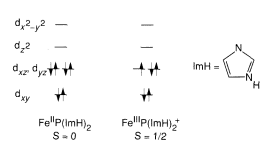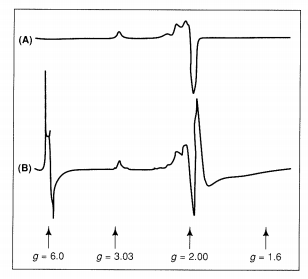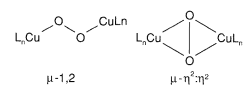# 5.4: Cytochrome c Oxidase

$$\newcommand{\vecs}{\overset { \rightharpoonup} {\mathbf{#1}} }$$ $$\newcommand{\vecd}{\overset{-\!-\!\rightharpoonup}{\vphantom{a}\smash {#1}}}$$$$\newcommand{\id}{\mathrm{id}}$$ $$\newcommand{\Span}{\mathrm{span}}$$ $$\newcommand{\kernel}{\mathrm{null}\,}$$ $$\newcommand{\range}{\mathrm{range}\,}$$ $$\newcommand{\RealPart}{\mathrm{Re}}$$ $$\newcommand{\ImaginaryPart}{\mathrm{Im}}$$ $$\newcommand{\Argument}{\mathrm{Arg}}$$ $$\newcommand{\norm}{\| #1 \|}$$ $$\newcommand{\inner}{\langle #1, #2 \rangle}$$ $$\newcommand{\Span}{\mathrm{span}}$$ $$\newcommand{\id}{\mathrm{id}}$$ $$\newcommand{\Span}{\mathrm{span}}$$ $$\newcommand{\kernel}{\mathrm{null}\,}$$ $$\newcommand{\range}{\mathrm{range}\,}$$ $$\newcommand{\RealPart}{\mathrm{Re}}$$ $$\newcommand{\ImaginaryPart}{\mathrm{Im}}$$ $$\newcommand{\Argument}{\mathrm{Arg}}$$ $$\newcommand{\norm}{\| #1 \|}$$ $$\newcommand{\inner}{\langle #1, #2 \rangle}$$ $$\newcommand{\Span}{\mathrm{span}}$$$$\newcommand{\AA}{\unicode[.8,0]{x212B}}$$

Most of the O2 consumed by aerobic organisms is used to produce energy in a process referred to as "oxidative phosphorylation," a series of reactions in which electron transport is coupled to the synthesis of ATP and in which the driving force for the reaction is provided by the four-electron oxidizing power of O2 (Reaction 5.1). (This subject is described in any standard text on biochemistry and will not be discussed in detail here.) The next to the last step in the electrontransport chain produces reduced cytochrome c, a water-soluble electron-transfer protein. Cytochrome c then transfers electrons to cytochrome c oxidase, where they are ultimately transferred to O2. (Electron-transfer reactions are discussed in Chapter 6.)

Cytochrome c oxidase is the terminal member of the respiratory chain in all animals and plants, aerobic yeasts, and some bacteria.44-46 This enzyme is always found associated with a membrane: the inner mitochondrial membrane in higher organisms or the cell membrane in bacteria. It is a large, complex, multisubunit enzyme whose characterization has been complicated by its size, by the fact that it is membrane-bound, and by the diversity of the four redox metal sites, i.e., two copper ions and two heme iron units, each of which is found in a different type of environment within the protein. Because of the complexity of this system and the absence of detailed structural information, spectroscopic studies of this enzyme and comparisons of spectral properties with O2-binding proteins and with model iron-porphyrin and copper complexes have been invaluable in its characterization.

## Spectroscopic Characterization

### 1. Models

Iron-porphyrin complexes of imidazole are a logical starting point in the search for appropriate spectroscopic models for heme centers in metalloproteins, since the histidyl imidazole side chain is the most common axial ligand bound to iron in such enzymes. Iron-porphyrin complexes with two axial imidazole ligands are known for both the ferrous and ferric oxidation states.47$$\tag{5.32}$$Figure 5.20). (C) Simulated EPR spectrum of a typical organic free radical with no hyperfine interaction. Note the narrow linewidth and a g value close to 2.00, i.e., near the g value for a free electron.

Monoimidazole complexes of iron porphyrins are also known for both the ferrous and the ferric oxidation states. The design of these model complexes has been more challenging than for six-coordinate complexes because of the high affinity of the five-coordinate complexes for a sixth ligand. In the ferrous complex, five coordination has been achieved by use of 2-methylimidazole ligands, as described in Chapter 4. The ferrous porphyrin binds a single 2-methylimidazole ligand, and, because the Fell center is raised out of the plane of the porphyrin ring, the 2-methyl substituent suffers minimal steric interactions with the porphyrin. However, the affinity of the five-coordinate complex for another 2-methylimidazole ligand is substantially lower, because the FeII must drop down into the plane of the porphyrin to form the six-coordinate complexes, in which case the 2-methyl substitutents on both axial ligands suffer severe steric interactions with the porphyrin.51 Using this approach, five-coordinate monoimidazole complexes can be prepared. They are coordinatively unsaturated, and will bind a second axial ligand, such as O2 and CO. They have been extensively studied as models for O2-binding heme proteins such as hemoglobin and myoglobin. Monoimidazole ferrous porphyrins thus designed are high-spin d6 with four unpaired electrons. They are even-spin systems and EPR spectra have not been observed.

Five-coordinate monoimidazole ferric-porphyrin complexes have also been prepared in solution52 by starting with a ferric porphyrin complex with a very poorly coordinating anion, e.g., FeIIIP(SbF6). Addition of one equivalent of imidazole results in formation of the five-coordinate monoimidazole complex (Reaction 5.33).

$Fe^{III}(TPP)(SbF_{6}) + ImH \rightarrow [Fe^{III}(TPP)(ImH)]^{+} + SbF_{6}^{-} \tag{5.33}$

When imidazole is added to ferric-porphyrin complexes of other anionic ligands, e.g., CI-, several equivalents of imidazole are required to displace the more strongly bound anionic ligand; consequently, only six-coordinate complexes are observed (Reaction 5.34).

$FE^{III}(TPP)Cl + 2 ImH \rightarrow [Fe^{III}(TPP)(ImH)_{2}]^{+} + Cl^{-} \tag{5.34}$

Monoimidazole ferric porphyrins are coordinatively unsaturated, readily bind a second axial ligand, and thus are appropriate models for methemoglobin or metmyoglobin. The five-coordinate complexes are high-spin d5, but usually become low-spin upon binding another axial ligand to become six-coordinate.

### 2. Spectroscopy of the Enzyme

The oxidized form of cytochrome c oxidase contains two CuII and two FeIII heme centers. It can be fully reduced to give a form of the enzyme containing two CuI and two FeII heme centers.44-46 The heme found in cytochrome c oxidase is different from that found in other heme proteins. It is heme a, closely related to heme b, which is found in hemoglobin, myoglobin, and cytochrome P-450, but has one of the vinyl groups replaced by a farnesyl substituent and one of the methyl groups replaced by a formyl substituent (see 5.35).

Each of the four metal centers has a different coordination environment appropriate to its function. Cytochrome a and CuA appear solely to carry out an electron-transfer function without interacting directly with dioxygen. Cytochrome a3 and CuB appear to be part of a binuclear center that acts as the site for dioxygen binding and reduction. A schematic describing the probable nature of these four metal sites within cytochrome oxidase is given in Figure 5.3 and a description of the evidence supporting the formulation of each center then follows.Figure 5.3 - Schematic representation of the metal centers in cytrochrome c oxidase.

Cytochrome a in both oxidation states has spectral characteristics that are entirely consistent with a low-spin ferric heme center with two axial imidazole ligands. In its oxidized form, it gives an EPR spectrum with g values44-46 similar to those obtained with model ferric-porphyrin complexes with two axial imidazole ligands 49,50 (see above, Section IV.B.1). Moreover, addition of cyanide anion to the oxidized enzyme or CO to the reduced enzyme does not perturb this center, indicating that cyanide does not bind to the heme, again consistent with a six-coordinate heme. The absence of ligand binding is characteristic of six-coordinate heme sites found in electron-transfer proteins and suggests strongly that cytochrome a functions as an electron-transfer center within cytochrome c oxidase.

CuA is also believed to act as an electron-transfer site. It has quite remarkable EPR spectroscopic characteristics, with g values at g = 2.18, 2.03, and 1.99, and no hyperfine splitting,44-46 resembling more an organic free radical than a typical Cull center (see Figures 5.2 and 5.4A). ENDOR studies of yeast cytochrome c oxidase containing 2H-cysteine or 15N-histidine (from yeast grown with the isotopically substituted amino acids) showed shifts relative to the unsubstituted enzyme, indicating that both of these ligands are bound to CuA.54 But the linear electric-field effect of CuA did not give the patterns characteristic of CuII-histidine complexes, indicating that the unpaired electron is not on the copper ion.55 The current hypothesis about this center is that copper is bonded in a highly covalent fashion to one, or more likely two, sulfur ligands, and that the unpaired electron density is principally on sulfur, i.e., [Cull--SR$$\leftrightarrow$$CuI- • SR]. Copper-thiolate model complexes with spectroscopic properties similar to CuA have never been synthesized, presumably because such complexes are unstable with respect to disulfide bond formation, i.e, 2 RS• $$\rightarrow$$ RS-SR. In the enzyme, RS• radicals are presumably constrained in such a way that they cannot couple to form disulfide bonds.Figure 5.4 - (A) EPR spectrum of oxidized cytochrome c oxidase. The signal at g = 2 is assigned to CuAII and the signal at g = 3 is assigned to cytochrome a in the low-spin ferric state. No signals attributable to the other two metal centers, i.e., cytochrome a3 and CuB, are observed (see text). (B) Spectrum obtained after addition of NO. Binding of NO to CUBII decouples the two metal ions, and thereby allows the high-spin ferric heme signal of cytochrome a3 at g = 6 to be observed. (From M. Brunori et at., Adv. lnorg. Biochem. 7 (1988), 93-153, adapted from Reference 56.)

The other heme center, cytochrome a3, does bind ligands such as cyanide to the FellI form and carbon monoxide to the Fell form, indicating that it is either five-coordinate or that it has a readily displaceable ligand. Reaction with CO, for example, produces spectral changes characteristic of a five-coordinate ferrous heme binding CO to give the six-coordinate carbonmonoxy product analogous to MbCO. The cytochrome a3 site is therefore an excellent candidate for O2 binding within cytochrome oxidase.

The EPR spectrum of fully oxidized cytochrome c oxidase might be expected to give signals corresponding to two CuII centers and two ferric heme centers. In fact, all that is observed in the EPR spectrum of the oxidized enzyme is the typical low-spin six-coordinate ferric heme spectrum due to cytochrome G and the EPR signal attributed to CuA (see Figure 5.4A). The fact that signals attributable to cytochrome a3 and CuB are not observed in the EPR spectrum led to the suggestion that these two metal centers are antiferromagnetically coupled.44-46 The measured magnetic susceptibility for the isolated enzyme was found to be consistent with this hypothesis, suggesting that these two metal centers consist of an S = $$\frac{1}{2}$$ CuII antiferromagnetically coupled through a bridging ligand to a high-spin S = $$\frac{5}{2}$$ FeIII to give an S = 2 binuclear unit.45 EXAFS measurements indicating a copper-iron separation of 3-4 Å as well as the strength of the magnetic coupling suggest that the metal ions are linked by a single-atom ligand bridge, but there is no general agreement as to the identity of this bridge.45,46

The cytochrome a3-CuB coupling can be disrupted by reduction of the individual metal centers. In this fashion, a g = 6 ESR signal can be seen for cytochrome a3 or g = 2.053, 2.109, and 2.278 signals for CuB. Nitric-oxide binding to CuB also decouples the metals, allowing the g = 6 signal to be seen56 (see Figure 5.4B). Mössbauer spectroscopy also indicates that cytochrome a3 is high-spin in the oxidized as well as the reduced state.57 ENDOR studies suggest that CuB has three nitrogens from imidazoles bound to it with water or hydroxide as a fourth ligand.58 Studies using 15N-labeled histidine in yeast have demonstrated that histidine is a ligand to cytochrome a3.59 All of these features have been incorporated into Figure 5.3.

## Mechanism of Dioxygen Reduction

### 1. Models

Before we consider the reactions of cytochrome c oxidase with dioxygen, it is instructive to review the reactions of dioxygen with iron porphyrins and copper complexes. Dioxygen reacts with ferrous-porphyrin complexes to make mononuclear dioxygen complexes (Reaction 5.36; see preceding chapter for discussion of this important reaction). Such dioxygen complexes react rapidly with another ferrous porphyrin, unless sterically prevented from doing so, to form binuclear peroxo-bridged complexes60,61 (Reaction 5.37). These peroxo complexes are stable at low temperature, but, when the temperature is raised, the O—O bond cleaves and two equivalents of an iron(IV) oxo complex are formed (Reaction 5.38). Subsequent reactions between the peroxo-bridged complex and the FeIV oxo complex produce the$$\mu$$-oxo dimer (see Reactions 5.39-5.40).

$3Fe^{II}(P) + 3O_{2} \rightarrow 3Fe(P)(O_{2}) \tag{5.36}$

$3Fe(P)(O_{2}) + 3Fe^{II}(P) \rightarrow 3(P)Fe^{III}—O—O—Fe^{III}(P) \tag{5.37}$

$(P)Fe^{III}—O—O—Fe^{III}(P) \rightarrow 2Fe^{IV}(P)(O) \tag{5.38}$

$2Fe^{IV}(P)(O) + 2(P)Fe^{III}—O—O—Fe^{III}(P) \rightarrow 2(P)Fe^{III}—O—Fe^{III}(P) + 2Fe(P)(O_{2}) \tag{5.39}$

$2Fe(P)(O_{2}) \rightarrow 2 Fe^{II}(P) + 2O_{2} \tag{5.40}$

$4Fe^{II}(P) + O_{2} \rightarrow 2(P)Fe^{III}—O—Fe^{III}(P) \tag{5.41}$

The reaction sequence (5.36) to (5.40) thus describes a four-electron reduction of O2 in which the final products, two oxide, O2-, ligands act as bridging ligands in binuclear ferric-porphyrin complexes (Reaction 5.41).

Copper(l) complexes similarly react with dioxygen to form peroxo-bridged binuclear complexes.62 Such complexes do not readily undergo O—O bond cleavage, apparently because the copper(III) oxidation state is not as readily attainable as the Fe(IV) oxidation state in an iron-porphyrin complex. Nevertheless, stable peroxo complexes of copper(II) have been difficult to obtain, because, as soon as it is formed, the peroxo complex either is protonated to give free hydrogen peroxide or is itself reduced by more copper(l) (Reactions 5.42 to 5.46).

$2Cu^{I} + O_{2} \rightarrow Cu^{II}—O—O—Cu^{II} \tag{5.42}$

$Cu^{II}—O—O—Cu^{II} + 2H^{+} \rightarrow 2 Cu^{II} + H_{2}O_{2} \tag{5.43}$

$2Cu^{I} + H_{2}O_{2} + 2H^{+} \rightarrow 2 Cu^{II} + 2 H_{2}O \tag{5.44}$

or Cu^{II}—O—O—Cu^{II} + 2 Cu^{I} + 4 H^{+} \rightarrow 4 Cu^{II} + 2 H_{2}O \tag{5.45}\]

$4 Cu^{I} + O_{2} + 4 H^{+} \rightarrow 4 Cu^{II} + 2 H_{2}O \tag{5.46}$

Recently, however, examples of the long-sought stable binuclear copper(II) peroxo complex have been successfully synthesized and characterized, and interestingly enough, two entirely different structural types have been identified, i.e., $$\mu$$-1,2 and $$\mu$$-$$\eta^{2}$$:$$\eta^{2}$$ dioxygen complexes63,64 (see 5.47)$$\tag{5.47}$$

### 2. Mechanistic Studies of the Enzyme

A single turnover in the reaction of cytochrome c oxidase involves (1) reduction of the four metal centers by four equivalents of reduced cytochrome c, (2) binding of dioxygen to the partially or fully reduced enzyme, (3) transfer of four electrons to dioxygen, coupled with (4) protonation by four equivalents of protons to produce two equivalents of water, all without the leakage of any substantial amount of potentially harmful partially reduced dioxygen byproducts such as superoxide or hydrogen peroxide.44-46 At low temperatures, the reaction can be slowed down, so that the individual steps in the dioxygen reduction can be observed. Such experiments are carried out using the fully reduced enzyme to which CO has been bound. Binding of CO to the Fell heme center in reduced cytochrome c oxidase inhibits the enzyme and makes it unreactive to dioxygen. The CO-inhibited derivative can then be mixed with dioxygen and the mixture cooled. Photolysis of metal-CO complexes almost always leads to dissociation of CO, and CO-inhibited cytochrome c oxidase is no exception. Photolytic dissociation of CO frees the Fell heme, thereby initiating the reaction with dioxygen, which can then be followed spectroscopically.44-46 Dioxygen reacts very rapidly with the fully reduced enzyme to produce a species that appears to be the dioxygen adduct of cytochrome a3 (Reaction 5.48). Such a species is presumed to be similar to other mononuclear oxyheme derivatives. The dioxygen ligand in this species is then rapidly reduced to peroxide by the nearby CuB, forming what is believed to be a binuclear $$\mu$$-peroxo species (Reaction 5.49). These steps represent a two-electron reduction of dioxygen to the peroxide level, and are entirely analogous to the model reactions discussed above (Reactions 5.36 to 5.46), except that the binuclear intermediates contain one copper and one heme iron. The $$\mu$$-peroxo FellI - (O22-) - Cull species is then reduced by a third electron, resulting in cleavage of the O—O bond (Reaction 5.50). One of the oxygen atoms remains with iron in the form of a ferryl complex, i. e., an FeIV oxo, and the other is protonated and bound to copper in the form of a CuII aquo complex.65 Reduction by another electron leads to hydroxo complexes of both the FeIII heme and the CuII centers (Reaction 5.51).65 Protonation then causes dissociation of two water molecules from the oxidized cytochrome a3-CuB center (Reaction 5.52).

$(cyt\; a_{3})\overbrace{Fe^{II} \quad Cu_{B}^{I}} + O_{2} \rightarrow (cyt\; a_{3})\overbrace{Fe^{III}(O_{2}^{-}) \quad Cu_{B}^{I}} \tag{5.48}$

$(cyt\; a_{3})\overbrace{Fe^{III}(O_{2}^{-}) \quad Cu_{B}^{I}} \rightarrow (cyt\; a_{3})\overbrace{Fe^{III}-(O_{2}^{2-}) \quad Cu_{B}^{II}} \tag{5.49}$

$(cyt\; a_{3})\overbrace{Fe^{III}-(O_{2}^{2-})-Cu_{B}^{II}} + e^{-} + 2H^{+} \rightarrow (cyt\; a_{3})\overbrace{Fe^{IV}=O \quad H_{2}O-Cu_{B}^{II}} \tag{5.50}$

$(cyt\; a_{3})\overbrace{Fe^{IV}=O \quad H_{2}O-Cu_{B}^{II}} +e^{-} \rightarrow (cyt\; a_{3})\overbrace{Fe^{III}-(OH^{-}) \quad (HO^{-})-Cu_{B}^{II}} \tag{5.51}$

$(cyt\; a_{3})\overbrace{Fe^{III}-(OH^{-}) \quad (HO^{-})-Cu_{B}^{II}} + 2H^{+} \rightarrow (cyt\; a_{3})\overbrace{Fe^{III} \quad Cu_{B}^{II}} + 2 H_{2}O \tag{5.52}$

Several important questions remain to be resolved in cytochrome c oxidase research. One is the nature of the ligand bridge that links cytochrome a3 and CuB in the oxidized enzyme. Several hypotheses have been advanced (imidazolate, thiolate sulfur, and various oxygen ligands), but then discarded or disputed, and there is consequently no general agreement concerning its identity. However, EXAFS measurements of metal-metal separation and the strength of the magnetic coupling between the two metal centers provide evidence that a single atom bridges the two metals.45,46 Another issue, which is of great importance, is to find out how the energy released in the reduction of dioxygen is coupled to the synthesis of ATP. It is known that this occurs by coupling the electron-transfer steps to a proton-pumping process, but the molecular mechanism is unknown.46 Future research should provide some interesting insights into the mechanism of this still mysterious process.

5.4: Cytochrome c Oxidase is shared under a CC BY-NC-SA 4.0 license and was authored, remixed, and/or curated by LibreTexts.